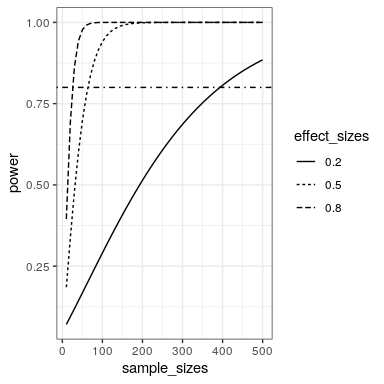# 19.2: Power Curves

We can also create plots that can show us how the power to find an effect varies as a function of effect size and sample size. We willl use the crossing() function from the tidyr package to help with this. This function takes in two vectors, and returns a tibble that contains all possible combinations of those values.

effect_sizes <- c(0.2, 0.5, 0.8)
sample_sizes = seq(10, 500, 10)

#
input_df <- crossing(effect_sizes,sample_sizes)
glimpse(input_df)
## Observations: 150
## Variables: 2
## $effect_sizes <dbl> 0.2, 0.2, 0.2, 0.2, 0.2, 0.2, 0.2, 0… ##$ sample_sizes <dbl> 10, 20, 30, 40, 50, 60, 70, 80, 90, …

Using this, we can then perform a power analysis for each combination of effect size and sample size to create our power curves. In this case, let’s say that we wish to perform a two-sample t-test.

# create a function get the power value and
# return as a tibble
get_power <- function(df){
power_result <- pwr.t.test(n=df$sample_sizes, d=df$effect_sizes,
type='two.sample')
df$power=power_result$power
return(df)
}

# run get_power for each combination of effect size
# and sample size

power_curves <- input_df %>%
do(get_power(.)) %>%
mutate(effect_sizes = as.factor(effect_sizes)) 

Now we can plot the power curves, using a separate line for each effect size.

ggplot(power_curves,
aes(x=sample_sizes,
y=power,
linetype=effect_sizes)) +
geom_line() +
geom_hline(yintercept = 0.8,
linetype='dotdash')